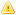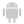Home |Sign in | EnglishHelpWLanguageWLanguage functionsControls, pages and windowsDrawing functionsPrefix syntaxDrawing functions on WDPic variablesDrawPolygonPresentationExampleCoordinatesDrawing with opacity or anti-aliasingRelated examplesSee alsoOpenSaveAlphaBlendBackgroundChangeModeDrawArcDrawChordDrawCircleDrawLineDrawPointDrawPolygonDrawPolylineDrawRectangleDrawRectangleGradientDrawRoundedRectangleDrawSliceDrawTextDrawTextRTFEndDrawingFillFontHorizontalSymmetryInvertColorMergeLayerPixelColorPixelOpacityResizeRotationSaveStartDrawingVerticalSymmetryPicMergeLayerPicOpenPicResizePicRotationPicSaveThis content has been translated automatically. Click here to view the French version.
• Coordinates
• Drawing with opacity or anti-aliasing
WINDEVWEBDEVWINDEV MobileOthers<WDPic variable>.DrawPolygon (Function)
In french: <Variable WDPic>.DessinePolygone
Draws a polygon in an WDPic variable.
Example
MonImageWDPic is WDPic = "Test.gif"
// Dessin d'un polygone à 4 côtés dont le
// fond est jaune clair et le bord bleu clair
MonImageWDPic.DrawPolygon(4, 10, 20, 20, 50, 40, 60, 50, 10, LightYellow, LightBlue)
IMG_MonDessin = MonImageWDPic
Syntax

Building a polygon point by point

<WDPic image>.DrawPolygon(<Number of sides> , <X1> , <Y1> , <X2> , <Y2> [, <X3> , <Y3> [... [, <Xn> , <Yn>]]] [, <Background color> [, <Side color>]])
<WDPic image>: WDPic variable
Name of the variable of type WDPic to be used. Only the background layer will be handled.
<Number of sides>: entier
Number of sides in the polygon (up to 23 sides). This parameter defines the number of <X>, <Y> pairs to specify in the function.
<X1>: entier
X-coordinate of first point of the polygon. These coordinates are expressed in pixels.
<Y1>: entier
Y-coordinate of first point of the polygon. These coordinates are expressed in pixels.
<X2>: entier
X-coordinate of second point of the polygon. These coordinates are expressed in pixels.
<Y2>: entier
Y-coordinate of second point of the polygon. These coordinates are expressed in pixels.
<X3>: entier
X-coordinate of 3rd point of the polygon. These coordinates are expressed in pixels.
<Y3>: entier
Y-coordinate of 3rd point of the polygon. These coordinates are expressed in pixels.
<Xn>: Optional integer
X coordinate of nth point of the polygon. These coordinates are expressed in pixels.
<Yn>: Optional integer
Y coordinate of nth point of the polygon. These coordinates are expressed in pixels.
<Background color>: Integer or constant (optional)
Polygon background color. This color can correspond to:
If this parameter is not specified, the background color:
<Side color>: Integer or constant (optional)
Color of polygon sides. This color can correspond to:
If this parameter is not specified, the line color:

Building a polygon via an array of X and Y-coordinates

<WDPic image>.DrawPolygon(<Array of coordinates> [, <Background color> [, <Side color>]])
<WDPic image>: WDPic variable
Name of the variable of type WDPic to be used. Only the background layer will be handled.
<Array of coordinates>: Array of integers or array of points
• WLanguage array of integers containing the coordinates of polygon points. The even subscript represent the X-coordinates while the odd subscripts represent the Y-coordinates of points. These coordinates are expressed in pixels.
•WLanguage array of Point variables containing the coordinates of polygon points. These coordinates are expressed in pixels.
<Background color>: Integer or constant (optional)
Polygon background color. This color can correspond to:
• an RGB color (returned by RGB),
• an HSL color (returned by HSL),
• a WLanguage preset color,
•a Variable of type Color .
If this parameter is not specified, the background color:
<Side color>: Integer or constant (optional)
Color of polygon sides. This color can correspond to:
• an RGB color (returned by RGB),
• an HSL color (returned by HSL),
• a WLanguage preset color,
•a variable of type Color.
If this parameter is not specified, the line color:Versions 26 and laterConstruction of a polygon from an Variable of the Polygon type

<WDPic image>.DrawPolygon(<Polygon> [, <Background color> [, <Side color>]])
<WDPic image>: WDPic variable
Name of the variable of type WDPic to be used. Only the background layer will be handled.
<Polygon>: Variable type Polygon
Name of the variable of type Polygon describing the different points of the Polygon.
<Background color>: Integer or constant (optional)
Polygon background color. This color can correspond to:
If this parameter is not specified, the background color:
<Side color>: Integer or constant (optional)
Color of polygon sides. This color can correspond to:
If this parameter is not specified, the line color:New in version 26Construction of a polygon from an Variable of the Polygon type

<WDPic image>.DrawPolygon(<Polygon> [, <Background color> [, <Side color>]])
<WDPic image>: WDPic variable
Name of the variable of type WDPic to be used. Only the background layer will be handled.
<Polygon>: Variable type Polygon
Name of the variable of type Polygon describing the different points of the Polygon.
<Background color>: Integer or constant (optional)
Polygon background color. This color can correspond to:
If this parameter is not specified, the background color:
<Side color>: Integer or constant (optional)
Color of polygon sides. This color can correspond to:
If this parameter is not specified, the line color:Construction of a polygon from an Variable of the Polygon type

<WDPic image>.DrawPolygon(<Polygon> [, <Background color> [, <Side color>]])
<WDPic image>: WDPic variable
Name of the variable of type WDPic to be used. Only the background layer will be handled.
<Polygon>: Variable type Polygon
Name of the variable of type Polygon describing the different points of the Polygon.
<Background color>: Integer or constant (optional)
Polygon background color. This color can correspond to:
If this parameter is not specified, the background color:
<Side color>: Integer or constant (optional)
Color of polygon sides. This color can correspond to:
If this parameter is not specified, the line color:
Remarks

Coordinates

Drawing a polygon:
MonImage is WDPic
MonImage.DrawPolygon(5, 80, 10, 10, 150, 100, 200, 160, 80, 140, 40)Coordinates are given with respect to the top left corner of the image (coordinates: (0.0)).
Important: There is no need to close the polygon (the coordinates of last point do not have to be equal to the coordinates of first point). The polygon is automatically "closed".
Limits: a polygon can contain up to 100 000 points in WINDEV, WEBDEV and Java, and up to 1000 points in Windows CE.Drawing with opacity or anti-aliasing

When drawing with opacity (<WDPic variable>.StartDrawing with the dWithOpacity constant) or with anti-aliasing (<WDPic variable>.ChangeMode with the drawAntiAliasing constant), the border is drawn above the inside of the polygon: therefore, the line is partly combined with the inside of the polygon. If the line is very thick, it will be displayed in two colors.
Related Examples:Unit examples (WINDEV): The drawing functions [ + ] Using the main drawing functions of WINDEV to:- Initialize an Image control for drawing- Draw simple shapes- Write a text into a drawing- Change the color in a drawing
Business / UI classification: Neutral code
Component: wd270pnt.dll
Minimum version required
• Version 25